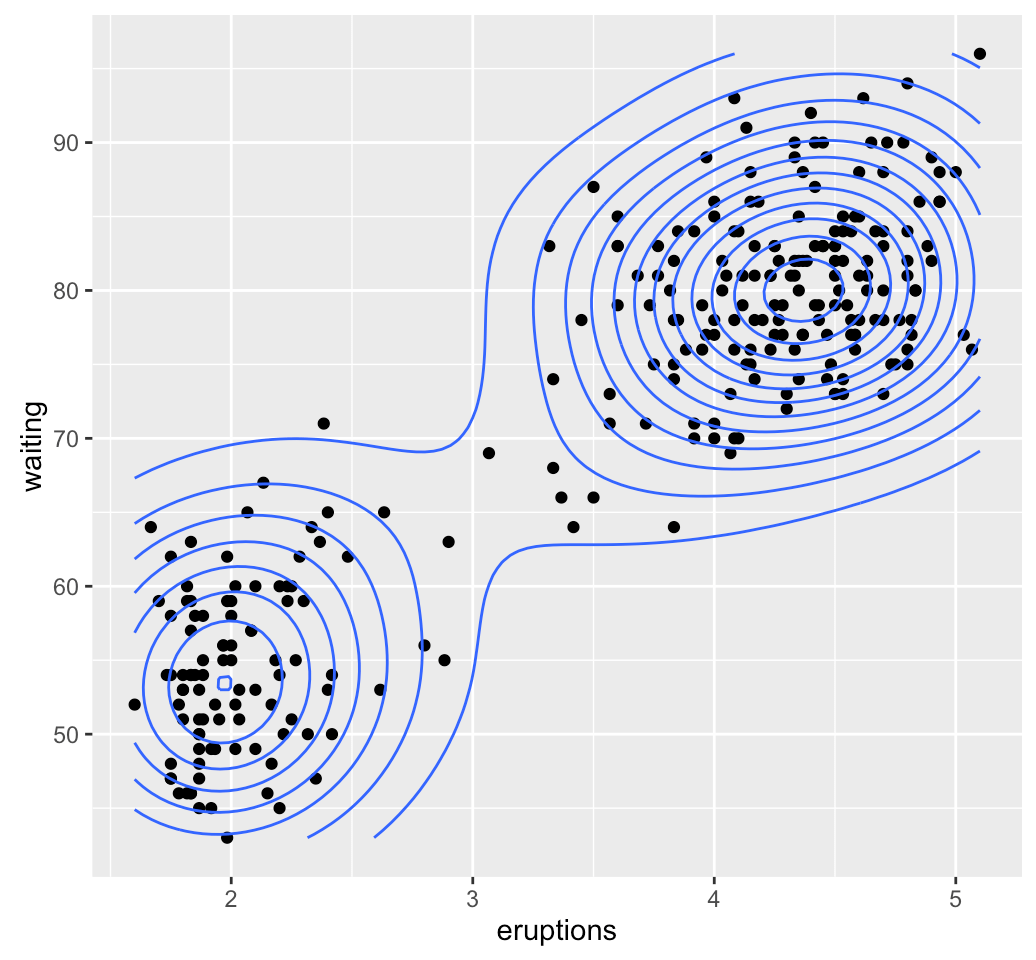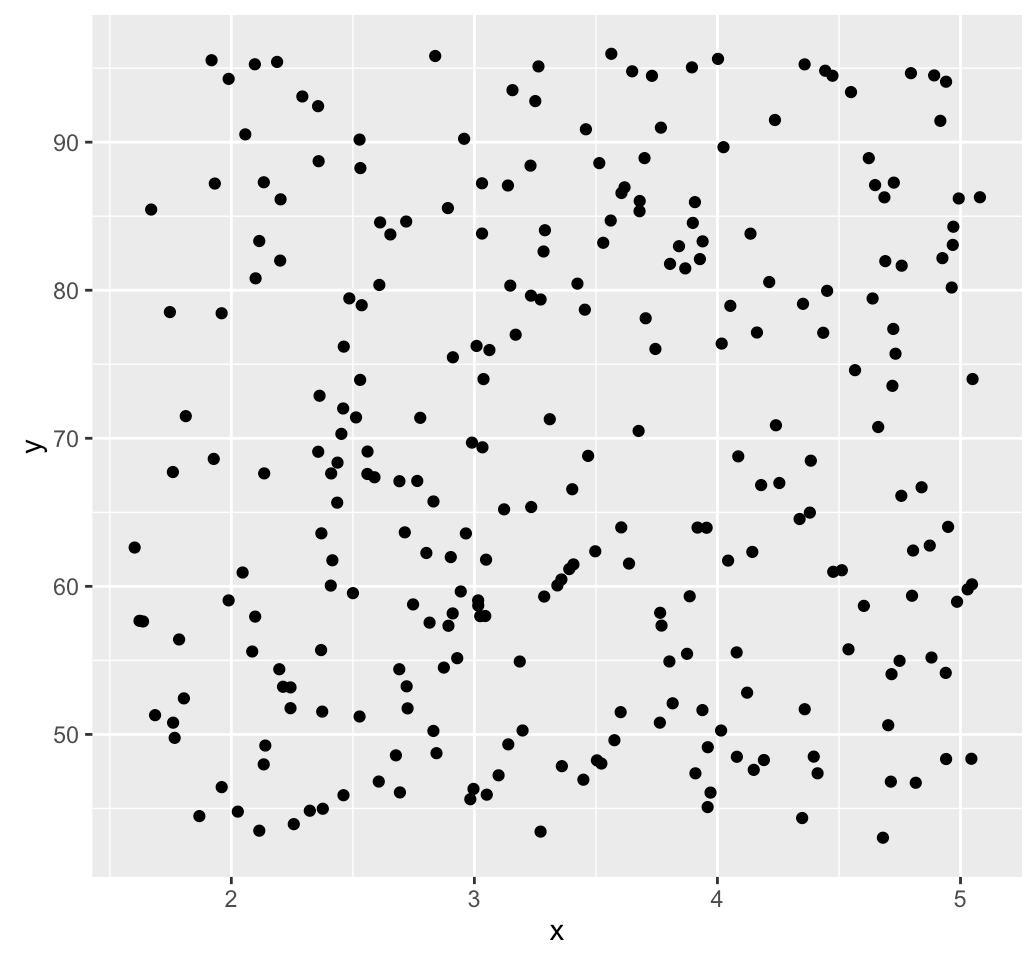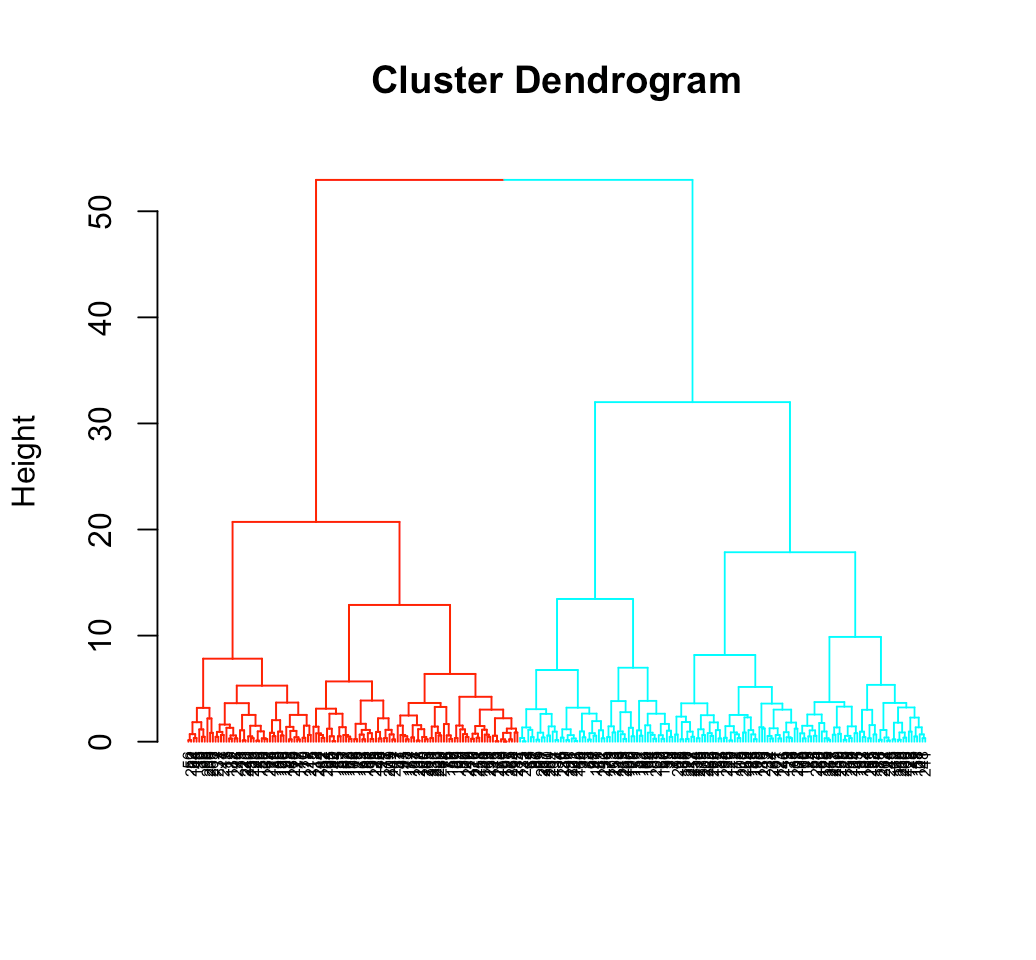Want to share your content on R-bloggers? click here if you have a blog, or here if you don't.

Clustering algorithms, including partitioning methods (K-means, PAM, CLARA and FANNY) and hierarchical clustering, are used to split the dataset into groups or clusters of similar objects.

Before applying any clustering method on the dataset, a natural question is:

Does the dataset contains any inherent clusters?

A big issue, in unsupervised machine learning, is that clustering methods will return clusters even if the data does not contain any clusters. In other words, if you blindly apply a clustering analysis on a dataset, it will divide the data into clusters because that is what it supposed to do.

Therefore before choosing a clustering approach, the analyst has to decide whether the dataset contains meaningful clusters (i.e nonrandom structures) or not. If yes, then how many clusters are there. This process is defined as the assessing of clustering tendency or the feasibility of the clustering analysis.

In this chapter:

• We describe why we should evaluate the clustering tendency (i.e., clusterability) before applying any cluster analysis on a dataset.
• We describe statistical and visual methods for assessing the clustering tendency
• R lab sections containing many examples are also provided for computing clustering tendency and visualizing clusters

# 1 Required packages

The following R packages are required in this chapter:

• factoextra for data visualization
• clustertend for assessing clustering tendency
• seriation for visually assessment of cluster tendency
1. factoextra can be installed as follow:
if(!require(devtools)) install.packages("devtools")
devtools::install_github("kassambara/factoextra")
1. Install clustertend and seriation:
install.packages("clustertend")
install.packages("seriation")
library(factoextra)
library(clustertend)
library(seriation)

# 2 Data preparation

We’ll use two datasets: the built-in R dataset faithful and a simulated dataset.

## 2.1 faithful dataset

faithful dataset contains the waiting time between eruptions and the duration of the eruption for the Old Faithful geyser in Yellowstone National Park (Wyoming, USA).

# Load the data
data("faithful")
df <- faithful
##   eruptions waiting
## 1     3.600      79
## 2     1.800      54
## 3     3.333      74
## 4     2.283      62
## 5     4.533      85
## 6     2.883      55

An illustration of the data can be drawn using ggplot2 package as follow:

library("ggplot2")
ggplot(df, aes(x=eruptions, y=waiting)) +
geom_point() +  # Scatter plot
geom_density_2d() # Add 2d density estimation## 2.2 Random uniformly distributed dataset

The R code below generates a random uniform data with the same dimension as the faithful dataset. The function runif(n, min, max) is used for generating uniform distribution on the interval from min to max.

# Generate random dataset
set.seed(123)
n <- nrow(df)

random_df <- data.frame(
x = runif(nrow(df), min(df$eruptions), max(df$eruptions)),
y = runif(nrow(df), min(df$waiting), max(df$waiting)))

# Plot the data
ggplot(random_df, aes(x, y)) + geom_point()Note that for a given real dataset, random uniform data can be generated in a single line function call as follow:

random_df <- apply(df, 2,
function(x, n){runif(n, min(x), (max(x)))}, n)

# 3 Why assessing clustering tendency?

As shown above, we know that faithful dataset contains 2 real clusters. However the randomly generated dataset doesn’t contain any meaningful clusters.

The R code below computes k-means clustering and/or hierarchical clustering on the two datasets. The function fviz_cluster() and fviz_dend() [in factoextra] will be used to visualize the results.

library(factoextra)
set.seed(123)
# K-means on faithful dataset
km.res1 <- kmeans(df, 2)
fviz_cluster(list(data = df, cluster = km.res1$cluster), frame.type = "norm", geom = "point", stand = FALSE)# K-means on the random dataset km.res2 <- kmeans(random_df, 2) fviz_cluster(list(data = random_df, cluster = km.res2$cluster),
frame.type = "norm", geom = "point", stand = FALSE)# Hierarchical clustering on the random dataset
fviz_dend(hclust(dist(random_df)), k = 2,  cex = 0.5)It can be seen that, k-means algorithm and hierarchical clustering impose a classification on the random uniformly distributed dataset even if there are no meaningful clusters present in it.

Clustering tendency assessment methods are used to avoid this issue.

# 4 Methods for assessing clustering tendency

Clustering tendency assessment determines whether a given dataset contains meaningful clusters (i.e., non-random structure).

In this section, we’ll describe two methods for determining the clustering tendency: i) a statistical (Hopkins statistic) and ii) a visual methods (Visual Assessment of cluster Tendency (VAT) algorithm).

## 4.1 Hopkins statistic

Hopkins statistic is used to assess the clustering tendency of a dataset by measuring the probability that a given dataset is generated by a uniform data distribution. In other words it tests the spatial randomness of the data.

### 4.1.1 Algorithm

Let D be a real dataset. The Hopkins statistic can be calculated as follow:

1. Sample uniformly $$n$$ points ($$p_1$$,…, $$p_n$$) from D.
2. For each point $$p_i \in D$$, find it’s nearest neighbor $$p_j$$; then compute the distance between $$p_i$$ and $$p_j$$ and denote it as $$x_i = dist(p_i, p_j)$$
3. Generate a simulated dataset ($$random_D$$) drawn from a random uniform distribution with $$n$$ points ($$q_1$$,…, $$q_n$$) and the same variation as the original real dataset D.
4. For each point $$q_i \in random_D$$, find it’s nearest neighbor $$q_j$$ in D; then compute the distance between $$q_i$$ and $$q_j$$ and denote it $$y_i = dist(q_i, q_j)$$
5. Calculate the Hopkins statistic (H) as the mean nearest neighbor distance in the random dataset divided by the sum of the mean nearest neighbor distances in the real and across the simulated dataset.

The formula is defined as follow:

$H = \frac{\sum\limits_{i=1}^ny_i}{\sum\limits_{i=1}^nx_i + \sum\limits_{i=1}^ny_i}$

A value of H about 0.5 means that $$\sum\limits_{i=1}^ny_i$$ and $$\sum\limits_{i=1}^nx_i$$ are close to each other, and thus the data D is uniformly distributed.

The null and the alternative hypotheses are defined as follow:

• Null hypothesis: the dataset D is uniformly distributed (i.e., no meaningful clusters)
• Alternative hypothesis: the dataset D is not uniformly distributed (i.e., contains meaningful clusters)

If the value of Hopkins statistic is close to zero, then we can reject the null hypothesis and conclude that the dataset D is significantly a clusterable data.

### 4.1.2 R function for computing Hopkins statistic

The function hopkins() [in clustertend package] can be used to statistically evaluate clustering tendency in R. The simplified format is:

hopkins(data, n, byrow = F, header = F)

• data: a data frame or matrix
• n: the number of points to be selected from the data
• byrow: logical value. If FALSE (default), the variables is taken by columns, otherwise the variables is taken by rows
• header: logical. If FALSE (the default) the first column (or row) will be deleted in the calculation

library(clustertend)
# Compute Hopkins statistic for faithful dataset
set.seed(123)
hopkins(faithful, n = nrow(faithful)-1)
## $H ##  0.1588201 # Compute Hopkins statistic for a random dataset set.seed(123) hopkins(random_df, n = nrow(random_df)-1) ##$H
##  0.5388899

It can be seen that faithful dataset is highly clusterable (the H value = 0.15 which is far below the threshold 0.5). However the random_df dataset is not clusterable ($$H = 0.53$$)

## 4.2 VAT: Visual Assessment of cluster Tendency

The visual assessment of cluster tendency (VAT) has been originally described by Bezdek and Hathaway (2002). This approach can be used to visually inspect the clustering tendency of the dataset.

### 4.2.1 VAT Algorithm

The algorithm of VAT is as follow:

1. Compute the dissimilarity (DM) matrix between the objects in the dataset using Euclidean distance measure
2. Reorder the DM so that similar objects are close to one another. This process create an ordered dissimilarity matrix (ODM)
3. The ODM is displayed as an ordered dissimilarity image (ODI), which is the visual output of VAT

### 4.2.2 R functions for VAT

We start by scaling the data using the function scale(). Next we compute the dissimilarity matrix between observations using the function dist(). finally the function dissplot() [in the package seriation] is used to display an ordered dissimilarity image.

The R code below computes VAT algorithm for the faithful dataset

library("seriation")
# faithful data: ordered dissimilarity image
df_scaled <- scale(faithful)
df_dist <- dist(df_scaled)
dissplot(df_dist)The gray level is proportional to the value of the dissimilarity between observations: pure black if $$dist(x_i, x_j) = 0$$ and pure white if $$dist(x_i, x_j) = 1$$. Objects belonging to the same cluster are displayed in consecutive order.

The VAT detects the clustering tendency in a visual form by counting the number of square shaped dark blocks along the diagonal in a VAT image.

The figure above suggests two clusters represented by two well-formed black blocks.

The same analysis can be done with the random dataset:

# faithful data: ordered dissimilarity image
random_df_scaled <- scale(random_df)
random_df_dist <- dist(random_df_scaled)
dissplot(random_df_dist)It can be seen that the random_df dataset doesn’t contain any evident clusters.

Now, we can perform k-means on faithful dataset and add cluster labels on the dissimilarity plot:

set.seed(123)
km.res <- kmeans(scale(faithful), 2)
dissplot(df_dist, labels = km.res$cluster)After showing that the data is clusterable, the next step is to determine the number of optimal clusters in the data. This will be described in the next chapter. # 5 A single function for Hopkins statistic and VAT The function get_clust_tendency() [in factoextra package] can be used to compute Hopkins statistic and provides also an ordered dissimilarity image using ggplot2, in a single function call. The ordering of dissimilarity matrix is done using hierarchical clustering. # Cluster tendency clustend <- get_clust_tendency(scale(faithful), 100) # Hopkins statistic clustend$hopkins_stat
##  0.1482683
# Customize the plot
clustend\$plot +
scale_fill_gradient(low = "steelblue", high = "white")# 6 Infos

This analysis has been performed using R software (ver. 3.2.4)